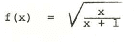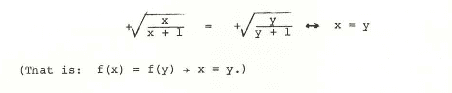# Proving an inverse function

ifthen to prove an inverse of this exists the following has been done to show that it is one to onewhat is the basis of equating the 2 square roots ?

Simon Bridge
Homework Helper
Can you think of another way to show it is 1-1?

Mark44
Mentor
if
View attachment 94299 then to prove an inverse of this exists the following has been done to show that it is one to one

View attachment 94298

what is the basis of equating the 2 square roots ?
What is the definition of a function being one-to-one?

What is the definition of a function being one-to-one?
an output can have only one input ,but what i don't understand is the basis of the expressionCan you think of another way to show it is 1-1?
yes i can reach the conclusion if we draw a graph but i'm confused about how to arrive at the conclusion (one to one ) using this data

jbriggs444
Homework Helper
yes i can reach the conclusion if we draw a graph but i'm confused about how to arrive at the conclusion (one to one ) using this data
If you graph it, can you think of a specific feature of the graph that you could phrase mathematically?

HallsofIvy
Homework Helper
an output can have only one input ,but what i don't understand is the basis of the expressionView attachment 94307
That is exactly what you said above: "an output can have only one input". If x and y are the "inputs" for the "outputs", f(x) and f(y), and they are the same, f(x)= f(y), so they are the same output, the inputs must be the same: x= y. Showing that "if f(x)= f(y) then x= y" is exactly the same as showing "an output can have only one input".

FactChecker
Gold Member
an output can have only one input ,but what i don't understand is the basis of the expressionView attachment 94307
It is the "If" part of an "if-then" statement. It is not proven, it is assumed. So there is no need for a "basis" for the statement.
If (x/(x+1))0.5 = (y/(y+1))0.5
then x=y.

This is all that you need to prove to show that the function has an inverse.

That is exactly what you said above: "an output can have only one input". If x and y are the "inputs" for the "outputs", f(x) and f(y), and they are the same, f(x)= f(y), so they are the same output, the inputs must be the same: x= y. Showing that "if f(x)= f(y) then x= y" is exactly the same as showing "an output can have only one input".
It is the "If" part of an "if-then" statement. It is not proven, it is assumed. So there is no need for a "basis" for the statement.
If (x/(x+1))0.5 = (y/(y+1))0.5
then x=y.

This is all that you need to prove to show that the function has an inverse.

so why have you proved an input equals an input ? when what we should prove is that for two particular inputs the OUTPUT will be the same only if those two inputs are equal.

Last edited by a moderator:
FactChecker